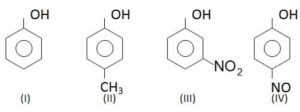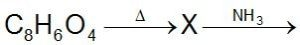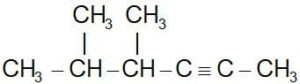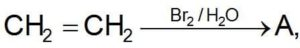# Question Bank for NEET Chemistry### National Eligibility Cum Entrance Test – NEET Chemistry Free Mock Test:

Online Tes for NEET Medical Entrance Exam on Chemistry single answer objective type questions as NEET / CBSE PMT exam pattern.

Ques. Reductive ozonolysis of benzene produces
(a) Acetone
(b) Maleic anhydride
(c) Phthalic acid
(d) Glyoxal

Ans. (d)

Ques. One mole of potassium dichromate completely oxidises the following number of moles of ferrous sulphate in acidic medium
(a) 1
(b) 3
(c) 5
(d) 6

Ans. (d)

Ques. The order of decreasing ease of reaction with ammonia is
(a) anhydrides, esters, ethers
(b) anhydrides, ethers, esters
(c) ethers, anhydrides, esters
(d) esters, ethers, anhydrides

Ans. (a)

Ques. Which electronic level would allow the hydrogen atom to absorb a photon but not to emit a photon
(a) 3s
(b) 2p
(c) 2s
(d) 1s

Ans. (d)

Ques. Which of the following elements will have the lowest electron affinity
(a) Nitrogen
(b) Flourine
(c) Chlorine
(d) Phosphorus

Ans. (d)

Related: Hydrogen and its compounds

Ques. The electrons identified by quantum numbers n and l (i) n = 4, l = 1 (ii) n = 4, l = 0 (iii) n = 3, l = 2 (iv) n = 3, l = 1 can be placed in order of increasing energy from the lowest to highest, as
(a) (iv) < (ii) < (iii) <(i)
(b) (ii) < (iv) < (i) < (iii)
(c) (i) < (iii) < (ii) < (iv)
(d) (i) < (iv) < (Ii) < (iii)

Ans. (a)

Ques. A catalyst ___
(a) Increases the average kinetic energy of reacting molecules
(b) Increases the activation energy
(c) Alters the reaction mechanism
(d) Increases the frequency of collisions of reacting species

Ans. (d)

Ques. The electronegativity of C, H, O, N and S are 2.5, 2.1, 3.5, 3.0 and 2.5 respectively. Which of the following bond is most polar
(a) O – H
(b) S – H
(c) N – H
(d) C – H

Ans. (a)

Ques. Normal aluminium electrode coupled with normal hydrogen electrode gives an  of 1.66 volts. So the standard electrode potential of aluminium is
(a) – 1.66 V
(b) + 1.66 V
(c) – 0.83 V
(d) + 0.83 V

Ans. (a)

Ques. In the reaction between ozone and hydrogen peroxide, H2O2 acts as
(a) Oxidising agent
(b) Reducing agent
(c) Bleaching agent
(d) Both oxidising and bleaching agent

Ans. (b)

Ques. The temperature coefficient of most of the reactions lies between
(a) 1 and 3
(b) 2 and 3
(c) 1 and 4
(d) 2 and 4

Ans. (b)

Ques. The oxidation state of Fe in K4[Fe(CN)6] is
(a) +2
(b) –2
(c) +3
(d) +4

Ans. (a)

Ques. The ability of a catalyst to accelerate the chemical reaction is known as
(a) Selectivelty
(b) Activity
(c) Negative catalyst
(d) None of these

Ans. (b)

Ques. The order of reactivity of halogen acids for reaction with C2H5OH is
(a) HCl > HBr > HI
(b) HI > HBr > HCl
(c) HBr > HI > HCl
(d) HBr > HCl > HI

Ans. (b)

Ques. Of the following substances the one which does not contain oxygen is
(a) Bauxite
(b) Epsom salt
(c) Cryolite
(d) Dolomite

Ans. (c)

Related: Biotechnology test questions

Ques. If 20 ml of methane (measured at room temperature) is burnt in 50 ml of oxygen, the volume of the gases left after cooling to room temperature will be
(a) 20 ml
(b) 30 ml
(c) 50 ml
(d) 70 ml

Ans. (b)

Ques. The most stable oxidation state of Mn is
(a) +2
(b) +4
(c) +5
(d) +7

Ans. (a)

Ques. Ligands in a complex are
(a) anions linked by coordinate bonds to a central metal atom or ion
(b) cations linked by coordinate bonds to a central metal atom or ion
(c) molecules linked by coordinate bonds to a central metal atom or ion
(d) ions or molecules linked by coordinate bonds to a central metal atom or ion

Ans. (d)

Ques. TiH1.73 is an example of
(a) Ionic hydride
(b) Covalent hydride
(c) Metallic hydride
(d) Polymeric hydride

Ans. (c)

Ques. What amount of ammonia is formed when 50 g each nitrogen and hydrogen react with each other?
(a) 50.0 g
(b) 100.0 g
(c) 34.0 g
(d) 60.7 g

Ans. (d)

Related: easy Trigonometry questions

Ques. Which of the following metals will not react with solution of CuSO4
(a) Fe
(b) Zn
(c) Mg
(d) Ag

Ans. (d)

Ques. What causes nitrogen to be chemically inert?
(a) Multiple bond formation in the molecule
(b) Absence of bond polarity
(c) Short internuclear distance
(d) High bond energy

Ans. (d)

Ques. Pb++, Cu++, Zn++, and Ni++ ions are present in a given acidic solution. On passing hydrogen sulphide gas through this solution the available precipitate will contain
(a) PbS and NiS
(b) PbS and CuS
(c) CuS and ZnS
(d) CuS and NiS

Ans. (b)

Ques. At 25o C temperature the 730 mm pressure, 380 ml of dry oxygen was collected. If the temperature in the field constant, what volume will the oxygen occupy at 760 mm pressure?
(a) 365 ml
(b) 449 ml
(c) 569 ml
(d) 621 ml

Ans. (a)

Ques. Nuclides
(a) Have specific atomic numbers
(b) Have same number of protons
(c) Have specific atomic number and mass numbers
(d) Are isotopes

Ans. (d)

Ques. Froth floatation process for the concentration of ores is an illustration of the practical application of
(b) absorption
(c) coagulation
(d) sedimentation

Ans. (a)

Ques. Which of the following undergoes nucleophilic substitution by SN1 mechanism
(a) Benzyl chloride
(b) Ethyl chloride
(c) Chlorobenzene
(d) Isopropyl chloride

Ans. (a)

Ques. Amorphous solids may also be classified as
(a) supercooled solids
(b) supercoolied liquids
(c) superheated solids
(d) superheated liquids

Ans. (b)

Ques. The total number of stereoisomeric forms of C6H6Cl6 known is
(a) 6
(b) 7
(c) 8
(d) 3

Ans. (c)

Ques. The element which has most stable +2 state
(a) C
(b) Si
(c) Sn
(d) None of these

Ans. (c)

Related: general Biology questions

Ques. 200 ml of phosphine decomposes to produce solid phosphorous and hydrogen. The change in volume is
(a) 100 ml increase
(b) 100 ml decrease
(c) 75 ml increase
(d) 75 ml decrease

Ans. (a)

Ques. The dimensions of pressure are the same as that of
(a) energy
(b) energy per unit volume
(c) force
(d) force per unit volume

Ans. (b)

Ques. How much of CaCO3 (in grams) should be decomposed to get enough CO2 which can convert 0.1 mole of Na2CO3 into NaHCO3?
(a) 100.0 g
(b) 20.0 g
(c) 10.0 g
(d) 5.0 g

Ans. (c)

Ques. Temporary hardness of water is due to the pressure of
(a) magnesium bicarbonate
(b) calcium chloride
(c) magnesium sulphate
(d) calcium carbonate

Ans. (a)

Ques. When the same amount of zinc is heated separately with an excess of H2SO4 and an excess of NaOH, the ratio of the volumes of hydrogen evolved is
(a) 1 : 1
(b) 1 : 2
(c) 2 : 1
(d) 3 : 2

Ans. (a)

Related: Nomenclature quiz chemistry

Ques. How many milliliters of 6.0 M HQ should be used to prepare 150 ml of a solution which is 0.3 M in hydrogen ion?
(a) 3.0
(b) 7.5
(c) 0.3
(d) –30

Ans. (b)

Ques. The temperature above which a gas remains ideal over a wide range of pressure is called
(a) boiling point
(b) Boyle’s temperature
(c) critical temperature
(d) ideal temperature

Ans. (b)

Ques. The standard enthalpy or the decomposition of N2O5 to NO2 is 58.04 kJ and standard entropy of this reaction is 176.7 J/K. The standard free energy change for this reaction at 25o C is
(a) –5.38 kJ
(b) 5.38 kJ
(c) 5.38 kJ
(d) –538 kJ

Ans. (d)

Ques. The co-ordination number of an atom or ion in solid is the
(a) number of nearest neighbours of that atom or ion
(b) oxidation number of that atom or ion
(c) formal charge on that atom or ion
(d) number of coordinate bonds formed with that atom or ion

Ans. (a)

Ques. How many grams of CaC2O4 will dissolve in distilled water to make one litre of saturated solution? (Solubility product of CaC2O4 is 2.5  10–9 mole2 litre–2 and its molecular weight is 128)
(a) 0.0064 gm
(b) 0.0128 gm
(c) 0.0032 gm
(d) 0.0640 gm

Ans. (a)

Related: Question Bank for Thermodynamics

Ques. A deuteron contains
(a) 1 neutron and 2 protons
(b) 1 neutron and 1 proton
(c) 1 neutron and 1 positron
(d) 2 neutrons and 1 proton

Ans. (b)

Ques. In the thermal dissociation of PCl5, the partial pressure in the gaseous equilibrium mixture is 1.0 atmosphere when half of PCl5 is found to dissociate. The equilibrium constant of the reaction (Kp) in atmosphere is
(a) 0.25
(b) 0.50
(c) 1.00
(d) 0.3

Ans. (d)

Ques. An ideal solution was obtained by mixing methanol and ethanol. If the partial vapour pressure of methanol and ethanol are 2.619 kPa and 4.556 kPa respectively, composition of the vapour (in terms of mole fraction) will be
(a) 0.365 methanol and 0.635 ethanol
(b) 0.635 methanol and 0.365 ethanol
(c) 0.50 methanol and 0.50 ethanol
(d) 0.173 methanol and 0.827 ethanol

Ans. (a)

Ques. Same mass of CH4 and H2 is taken in container. The partial pressure caused by H2 is
(a) 8 / 9
(b) 1 / 9
(c) 1 / 2
(d) 1

Ans. (a)

Ques. If 60% of the first order reaction was completed in 60 minutes, of the (given log 4 = 0.60; log 5 = 0.69) same reaction would be completed in approximately
(a) 40 minutes
(b) 50 minutes
(c) 45 minutes
(d) 60 minutes

Ans. (c)

Ques. The coordination number of a cation occupying a tetrahedral hole is
(a) 6
(b) 8
(c) 12
(d) 4

Ans. (d)

Ques. An example of a salt dissolved in water to give acidic solution is
(a) ammonium chloride
(b) sodium acetate
(c) potassium nitrate
(d) barium bromide

Ans. (a)

Ques. Wax is an example of
(a) Ionic crystal
(b) Covalent crystal
(c) Metallic crystal
(d) Molecular crystal

Ans. (d)

Ques. To a mixture of acetic acid and sodium acetate a further amount of sodium acetate is added. Then pH of the mixture
(a) increases
(b) decreases
(c) is unaltered
(d) unpredictable

Ans. (a)

Ques. The boiling point of a solution of 0.11 gm of a substance in 15 gm of ether was found to be 0.1o C higher than that of the pure ether. The molecular weight of the substance will be (Kb = 2.16)
(a) 148
(b) 158
(c) 168
(d) 178

Ans. (b)

Ques. At 100o C, Kw = 10–12. pH of pure water at 100o C will be:
(a) 7.0
(b) 6.0
(c) 8.0
(d) 12.0

Ans. (b)

Ques. Which of the following compound gives dye test
(a) Aniline
(b) Methylamine
(c) Diphenylamine
(d) Ethylamine

Ans. (a)

Ques. According to Le-Chatelier’s principle, adding heat to a solid and liquid in equilibrium will cause the
(a) amount of solid to decrease
(b) amount of liquid to decrease
(c) temperature to rise
(d) temperature to fall

Ans. (a)

Ques. A solution of a salt of a metal was electrolysed for 150 minutes with a current of 0.15 amperes. The weight of metal deposited was 0.783 gm. The equivalent weight of the metal is
(a) 55.97 gm
(b) 65.97 gm
(c) 75.97 gm
(d) 85.97 gm

Ans. (a)

Ques. What causes nitrogen to be chemically inert?
(a) Multiple bond formation in the molecule
(b) Absence of bond polarity
(c) Short internuclear distance
(d) High bond energy

Ans. (d)

Ques. The amount of KMnO4 required to prepare 100 ml of 0.1 N solution in alkaline medium is
(a) 1.58 gm
(b) 3.16 gm
(c) 0.52 gm
(d) 0.31 gm

Ans. (a)

Ques. At 500 K, the half life period of a gascons reaction at an initial pressure of 80 K Pa is 350 s. When the pressure is 40 K Pa, the half life period is 175 s, the order of the reaction is
(a) zero
(b) one
(c) two
(d) cannot be predicted

Ans. (a)

Ques. A radioactive element has a half-life of 20 minutes. How much time should elaspe before the element is reduced to 1/8 th of the original mass
(a) 40 minutes
(b) 60 minutes
(c) 80 minutes
(d) 160 minutes

Ans. (d)

Ques. Which of the following is an extensive property
(a) temperature
(b) chemical potential
(c) Gibb’s free energy
(d) Molar volume

Ans. (c)

Ques. If concentration of reactants is increased by ‘x’, then K becomes
(a) ln (K/x)
(b) K/x
(c) K + x
(d) K

Ans. (d)

Ques. The Langmuir adsorption isotherm is deduced using the assumption
(a) the adsorbed molecules interact with each other
(b) the adsorption take place in multi layers
(c) the adsorption sites are equivalent in their ability to absorb the particles
(d) the heat of adsorption varies with coverage

Ans. (c)

Ques. What is the entropy change (in JK–1 mol–1) when one mole of ice is converted into water at 0o C (The enthalpy change for the conversion of ice to liquid water is 6.0 kJ mol–1 at 0oC)
(a) 21.98
(b) 20.13
(c) 2.013
(d) 2.198

Ans. (a)

Ques. The efficiency of enzyme catalys is due to its capacity to
(a) form a strong enzyme-substrate complex
(b) change the shape of the substrate
(c) form a colloidal solution in water
(d) decrease the bond energies in substrate molecules

Ans. (a)

Ques. If the average velocity of N2 molecule is 0.3 m/s at 27o C, then the velocity will be 0.6 m/s at
(a) 1200 K
(b) 600 K
(c) 400 K
(d) 1800 K

Ans. (d)

Ques. Which of the following sets of quantum numbers represents the highest energy of an atom?
(a) n = 3, l = 0, m = 0, s = + 1/2
(b) n = 3, l = 1, m = 1, s = + 1/2
(c) n = 3, l = 2, m = 1, s = + 1/2
(d) n = 4, l = 0, m = 0, s = + 1/2

Ans. (c)

Ques. The pH of the solution: 5mL of M/5, HCl + 10 mL of M/10 NaOH is
(a) 5
(b) 3
(c) 7
(d) 8

Ans. (c)

Ques. An aqueous solution of FeCl3 (formula mass = 162) has a density of 1.1 g ml–1 and contains 20% FeCl3. Molarity of this solution is
(a) 0.028
(b) 0.163
(c) 0.2
(d) 1.27

Ans. (d)

Ques. In a photochemical reaction, the ratio of number of dissociate molecules and number of quanta of absorbed energy is called
(a) Einstein
(b) Quantum efficiency
(c) Quantum constant
(d) Planck constant

Ans. (b)

Ques. The most common lanthanide is
(a) Lanthanum
(b) Cerium
(c) Samarium
(d) Plutonium

Ans. (b)

Ques. The lightest metal is
(a) Li
(b) Cl
(c) Ca
(d) Na

Ans. (a)

Ques. A mixture of sand and iodine can be separated by
(a) Crystallisation
(b) Sublimation
(c) Distillation
(d) Fractional distillation

Ans. (b)

Ques. Nature of the bond formed between two elements depends on the
(a) Oxidation potential
(b) Electronegativity
(c) Ionization potential
(d) Electron affinity

Ans. (b)

Ques. Aldehydes are produced in atmosphere by
(a) Oxidation of secondary alcohols
(b) Reduction of alkenes
(c) Reaction of oxygen atoms with hydrocarbons
(d) Reaction of oxygen atoms with ozone

Ans. (c)

Ques. Which functional group participates in disulphide bond formation in proteins
(a) Thiolactone
(b) Thiol
(c) Thioether
(d) Thioester

Ans. (b)

Ques. The common acid used in the manufacture of rayon and plastics is
(a) Methanoic acid
(b) Ethanoic acid
(c) Propanoic acid
(d) Butanoic acid

Ans. (b)

Ques. In the following compoundsThe order of acidity is

(a) III > IV > I > II
(b) I > IV > III > II
(c) II > I > III > IV
(d) IV > III > I > II

Ans. (d)

Ques. In the reactionThe compound X is

(a) Phthalic anhydride
(b) Phthalic acid
(c) o-xylene
(d) Benzoic acid

Ans. (a)

Ques. Choose the correct IUPAC name of the compound(a) 2, 3-dimethyl-4-hexyne
(b) 4,5-dimethyl-2-hexyne
(c) 5-propyl-2-pentyne
(d) 2-propyl-3-pentyne

Ans. (b)

Ques. In the below reaction the compound A is(a) Ethylene bromohydrin
(b) 1, 2-dibromo ethane
(c) Ethanol
(d) None of these

Ans. (a)